## Check if the values of multiple columns of one dataset are in multiple columns of another dataset

I have columns HAC1 to HAC14 in df1 and columns DX1 to DX10 in df2. I do not want to merge df1 and df2 together. I want to check if any value from any rows of the column HAC1 is present in Row 1 of the columns DX1 to DX10. Create a new column called H1 in df2 and assign the value as 1 if any value from any rows of the column HAC1 is present in Row 1 of the columns DX1 to DX10 and 0 if the value is absent and go to HAC2. Check if any value from any rows of the column HAC2 is present in Row 1 of the columns DX1 to DX10. Create a new column called H2 in df2 and assign the value as 1 if any value from any rows of the column HAC2 is present in Row 1 of the columns DX1 to DX10 and 0 if the value is absent and go to HAC3. Continue the same process till HAC14.Then repeat the entire process for Row 2 of columns DX1 to DX10 and keep adding 1 or 0 to the created variables H1 to H14. Continue the entire process for n rows of df2. I have tried this: data df2; set df2; array H{14} H1-H14; do i =1 to 14; H{i} =0; end; do index =1 to _n_; flag =0; set df1; array HAC{14} HAC1-HAC14; do i=1 to 14; set df2; array DX{10} DX1-DX10; do j =1 to 10; if DX{j}=HAC{i} then do; flag=1; leave; end; end; H{i}=flag; end; output; end; run;
1 ACCEPTED SOLUTION

Accepted SolutionsKsharp
Super User

## Re: Check if the values of multiple columns of one dataset are in multiple columns of another datase

``````data df1;
input (HAC1 HAC2 HAC3 HAC14) (\$);
cards;
T81500A T800XXA L89003 I2602
T81501A  NaN    L89004 I2692
T81502A  NaN    NaN    182401
NaN      NaN     NaN   12699
;

data df2;
input (DX1 DX2 DX10) (\$);
cards;
I639    L89004  12602
T81500A T81502A R0789
I2692   I2602   Z8541
G8321   L89004  110
;

/*The first way is using Hash Table*/
data want;
if _n_=1 then do;
if 0 then set df1;
declare hash ha1(dataset:'df1');
ha1.definekey('HAC1');
ha1.definedone();
declare hash ha2(dataset:'df1');
ha2.definekey('HAC2');
ha2.definedone();
declare hash ha3(dataset:'df1');
ha3.definekey('HAC3');
ha3.definedone();
declare hash ha14(dataset:'df1');
ha14.definekey('HAC14');
ha14.definedone();
end;
set df2;
array h{*} h1 h2 h3 h14;
do i=1 to dim(h);
h{i}=0;
end;
array d{*} \$ DX1 DX2 DX10;
do i=1 to dim(d);
if ha1.check(key:d{i})=0 then h1+1;
if ha2.check(key:d{i})=0 then h2+1;
if ha3.check(key:d{i})=0 then h3+1;
if ha14.check(key:d{i})=0 then h14+1;
end;
drop i HAC1 HAC2 HAC3 HAC14;
run;``````

``````
/*The second way is using SAS/IML*/
proc iml;
use df1;
read all var _all_ into df1[c=vname1];
close;
use df2;
read all var _all_ into df2[c=vname2];
close;
h1=j(nrow(df2),1,0);
h2=j(nrow(df2),1,0);
h3=j(nrow(df2),1,0);
h14=j(nrow(df2),1,0);
do i=1 to nrow(df2);
h1[i]=sum(element(df2[i,],df1[,1]));
h2[i]=sum(element(df2[i,],df1[,2]));
h3[i]=sum(element(df2[i,],df1[,3]));
h14[i]=sum(element(df2[i,],df1[,4]));
end;
create temp var {h1 h2 h3 h14};
append;
close;
quit;
data want;
merge df2 temp;
run;``````
16 REPLIES 16

## Re: Check if the values of multiple columns of one dataset are in multiple columns of another datase

SAS uses variables to reference things. You cannot "add variables" to a data set. That change replaces a data set and best not to try as you can lose the data you have.

How do you expect to do something with two data sets without some sort of combining them?

Since you used code like this you have already altered your data Df2 and may not be at all representative of your original data set. Multiple SET statements have complex interactions.

```data df2;
set df2;
array H{14} H1-H14;
do i =1 to 14;
H{i} =0;
end;
do index =1 to _n_;
flag =0;
set df1;
array HAC{14} HAC1-HAC14;
do i=1 to 14;
set df2;
array DX{10} DX1-DX10;
do j =1 to 10;
if DX{j}=HAC{i} then do;
flag=1;
leave;
end;
end;
H{i}=flag;
end;
output;
end;
run;
```

When developing code it best not to use the same data set name as the result data as one of the sets on a Set or Merge statement as that 'new' set completely replaces the old one.

Example data of your two sets, best in the form of working data step code. Then show the result for that example data.

There are special functions for addressing specific observations in a data set. These would involve OPEN, POINT , FETCHOBS, CLOSE and possibly others.

## Re: Check if the values of multiple columns of one dataset are in multiple columns of another datase

Yes, I understand. I was able to achieve the expected output using python. I am looking for an alternative in SAS. I am new to SAS. Considering I create those variables in a new dataset, how can I achieve expected output. I appreciate your help.

df2[['H1', 'H2', 'H3', 'H4', 'H5', 'H6', 'H7', 'H8', 'H9', 'H10', 'H11', 'H12', 'H13', 'H14']]=0

for i in range(1,15):
for index,row in df2.iterrows():
flag =0
for j in range(1,11):
if row[f'DX{j}'] in df1[f'HAC{i}'].values:

flag =flag+1
print(flag)
#break
df2.at[index, f'H{i}'] = flag

## Re: Check if the values of multiple columns of one dataset are in multiple columns of another datase

Can you post an example of the data you have, with perhaps 5 rows of data in df1 with vars HAC1-HAC3 and 5 rows of data in df2 with vars DX1-DX3?  Posting it as SAS data step code with a CARDS statement will give people something to work with. And then also show the desired output.

Assuming DX1-DX10 are diagnostic codes, does that mean all the variables are character?

Roughly how many distinct values are there for for the diagnostic codes in df1?

Assuming you're a python person can you post the pandas code you would use to do this?  (I'm just curious).

It seems like a look-up table problem.  My first thought would be to create a SAS format or hash table (both similar to python dictionaries).

Check out the Boston Area SAS Users Group (BASUG) video archives: https://www.basug.org/videos.

## Re: Check if the values of multiple columns of one dataset are in multiple columns of another datase

Example df1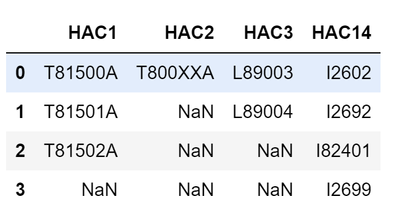Example df2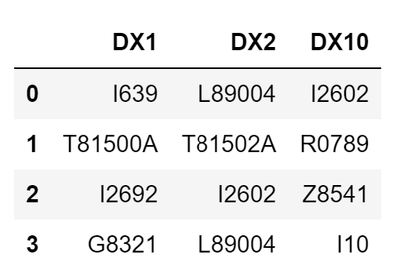Expected Output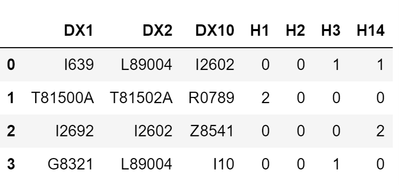Python Code: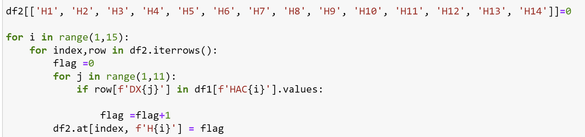## Re: Check if the values of multiple columns of one dataset are in multiple columns of another datase

Forget ROWS and COLUMNS.  Datasets have VARIABLES.  A dataset consists of multiple OBSERVATIONS.

Sounds like you have a set of patient data (your first dataset) and set of condition or procedure codes (your second dataset) and you to combine them to check if the patient has any of the conditions.

So if you have data like:

``````data patients;
input id (HAC1-HAC4) (:\$5.) ;
cards;
1 A B C .
2 D E . .
3 F . . .
4 A B D F
;

data codes ;
input disease \$ (DX1-DX3) (:\$5.);
cards;
HF A D .
DIAB C B F
;

``````

So you can use the POINT= option on the SET statement to re-read the CODES dataset multiple times.

``````data want;
set patients;
array hac hac1-hac4 ;
do cindex=1 to nobs;
set codes point=cindex nobs=nobs;
flag=0;
do hindex=1 to dim(hac) while(not missing(hac[hindex]) and not flag);
flag=0<whichc(hac[hindex], of dx1-dx3);
end;
output;
end;
drop hindex ;
run;``````

Result

```Obs   id   HAC1   HAC2   HAC3   HAC4   disease   DX1   DX2   DX3   flag

1     1    A      B      C             HF        A     D            1
2     1    A      B      C             DIAB      C     B     F      1
3     2    D      E                    HF        A     D            1
4     2    D      E                    DIAB      C     B     F      0
5     3    F                           HF        A     D            0
6     3    F                           DIAB      C     B     F      1
7     4    A      B      D      F      HF        A     D            1
8     4    A      B      D      F      DIAB      C     B     F      1
```

If you would prefer a WIDE format then perhaps just transpose the data.

``````proc transpose data=want out=wide(drop=_name_) prefix=dx_ ;
by id ;
id disease;
var flag;
run;``````

Result

``````Obs    id    dx_HF    dx_DIAB

1      1      1         1
2      2      1         0
3      3      0         1
4      4      1         1

``````

## Re: Check if the values of multiple columns of one dataset are in multiple columns of another datase

I appreciate your help. I am not exactly checking the presence of conditions. Can you please refer the example datasets and output I posted? Thank you so much!

## Re: Check if the values of multiple columns of one dataset are in multiple columns of another datase

I cannot program from photographs of data.

You don't explain how your example is different than mine.

So let's me guess.

1) You have MISSING values in some of the HACxxx variables (what your picture seems to be presenting as the string NaN) but you have not consolidated all of the non-missing values into the beginning of the list of values.  SO you will need to adjust how you handle those.  The code I posted would stop at the first missing value.

2) You seem to be COUNTING the matches instead of just FLAGGING them since your flag variables do not just have binary 0 or 1 values. Again you just need to adjust how you stop the looping and also what you do when a match is found.

If my guess are not right then explain what you are doing (not how the python code is doing it, but actually describe the analysis you are performing).

If you need more help then post the data as code. And post the code you tried and explain how it does not generate the result.  If you get error messages then post the log from the data step so we can see what code is generating what errors.

## Re: Check if the values of multiple columns of one dataset are in multiple columns of another datase

Note if the goal is just to count then convert your data in normalized structures and it will be much easier.

``````data patients;
infile cards truncover;
input id @ ;
do index=1 to 4;
input hac :\$5. @;
output;
end;
cards;
1 A B C .
2 D E . .
3 F . . .
4 A B D F
;

data codes ;
infile cards truncover;
input disease \$ @;
do index=1 to 3;
input dx :\$5. @ ;
output;
end;
cards;
HF A D .
DIAB C B F
;

proc sql;
create table TALL as
select a.id,b.disease
, sum(a.hac = b.dx and not missing(a.hac)) as flag
from patients a
, codes b
group by 1,2
;
quit;

proc transpose data=tall out=wide(drop=_name_);
by id;
id disease ;
var flag ;
run;

proc print;
run;
``````

Results

```Obs    id    DIAB    HF

1      1      2      1
2      2      0      1
3      3      1      0
4      4      2      2
```Ksharp
Super User

## Re: Check if the values of multiple columns of one dataset are in multiple columns of another datase

``````data df1;
input (HAC1 HAC2 HAC3 HAC14) (\$);
cards;
T81500A T800XXA L89003 I2602
T81501A  NaN    L89004 I2692
T81502A  NaN    NaN    182401
NaN      NaN     NaN   12699
;

data df2;
input (DX1 DX2 DX10) (\$);
cards;
I639    L89004  12602
T81500A T81502A R0789
I2692   I2602   Z8541
G8321   L89004  110
;

/*The first way is using Hash Table*/
data want;
if _n_=1 then do;
if 0 then set df1;
declare hash ha1(dataset:'df1');
ha1.definekey('HAC1');
ha1.definedone();
declare hash ha2(dataset:'df1');
ha2.definekey('HAC2');
ha2.definedone();
declare hash ha3(dataset:'df1');
ha3.definekey('HAC3');
ha3.definedone();
declare hash ha14(dataset:'df1');
ha14.definekey('HAC14');
ha14.definedone();
end;
set df2;
array h{*} h1 h2 h3 h14;
do i=1 to dim(h);
h{i}=0;
end;
array d{*} \$ DX1 DX2 DX10;
do i=1 to dim(d);
if ha1.check(key:d{i})=0 then h1+1;
if ha2.check(key:d{i})=0 then h2+1;
if ha3.check(key:d{i})=0 then h3+1;
if ha14.check(key:d{i})=0 then h14+1;
end;
drop i HAC1 HAC2 HAC3 HAC14;
run;``````

``````
/*The second way is using SAS/IML*/
proc iml;
use df1;
read all var _all_ into df1[c=vname1];
close;
use df2;
read all var _all_ into df2[c=vname2];
close;
h1=j(nrow(df2),1,0);
h2=j(nrow(df2),1,0);
h3=j(nrow(df2),1,0);
h14=j(nrow(df2),1,0);
do i=1 to nrow(df2);
h1[i]=sum(element(df2[i,],df1[,1]));
h2[i]=sum(element(df2[i,],df1[,2]));
h3[i]=sum(element(df2[i,],df1[,3]));
h14[i]=sum(element(df2[i,],df1[,4]));
end;
create temp var {h1 h2 h3 h14};
append;
close;
quit;
data want;
merge df2 temp;
run;``````

## Re: Check if the values of multiple columns of one dataset are in multiple columns of another datase

I think @Ksharp 's hash solution is cleaner than my below informat approach, but wanted to share a format solution since you're new to SAS.  A simple SAS informat is like a python dict, it holds a set of key-value pairs that can be used for lookups.  So you can load your codes into an informat, then use the informat to look up the diagnostic group for each dx code.

To create the informat:

``````data codes ;
input hac1 : \$12. hac2 : \$12. hac3 : \$12. hac4 : \$12.;
cards ;
T81500A T800XXA L89003 I2602
T81501A .       L89004 I2692
T81502A .       .      I82401
.       .       .      I2699
;

*Lookup table maps each code to 1-4 for hac1-hac4 ;
data codesvert (keep=hacno code fmtname type hlo rename=(code=start hacno=label ));
set codes end=last;
retain fmtname "hac" type "I" ;
array hac{*} hac1 hac2 hac3 hac4 ;

do i=1 to dim(hac) ;
hacno=i ;
code=hac{i} ;
if not missing(code) then output ;
end ;

if last then do ;
HLO='O' ;
code='' ;
hacno=. ;
output ;
end ;
run ;

proc print data=codesvert ;
run ;

*build an informat named hac, like a python dict, this simple one has key-value pairs ;

proc format cntlin=codesvert library=work fmtlib ;
run ;
``````

Once you have the informat built, you can use it like:

``````data dx ;
input dx1 : \$12. dx2 : \$12. dx3 : \$12.;
cards ;
I639    L89004  I2602
T81500A T81502A R0789
I2692   I2602   Z8541
G8321   L89004  I10
;

data want ;
set dx ;
array dx{*} dx1-dx3 ;
array h{4} ;
do i=1 to dim(h) ;
h{i}=0 ;
end ;
do i=1 to dim(dx) ;
*INPUT function uses the hac informat as a lookup table to look up the value 1-4 ;
if not missing (input(dx{i},hac.)) then h{input(dx{i},hac.)}+1 ;
end ;
drop i ;
run ;

proc print data=want ;
run ;
``````

Check out the Boston Area SAS Users Group (BASUG) video archives: https://www.basug.org/videos.

## Re: Check if the values of multiple columns of one dataset are in multiple columns of another datase

Hey, thank you so much for this. The second way (proc iml) is working without any errors and giving the expected output. The first way is giving an error saying argument is not of same length.

Also, I was wondering how to do the same if I had multiple conditions to check. For example, consider that I have variables Proc1 to Proc5 in df2 along with DX1 to DX10. I have P6 to P10 in df1 along with HAC1 to HAC14. Proc1 to Proc5 and P6 to P10 are both alphanumeric procedures codes.

For counting values under H1, we are checking if any value of HAC1 is present in DX1 to DX10. We do the same thing till H5.

For H6, there are conditions, if there is any value from P6 present in Proc1 to Proc5 AND there is any value from HAC6 present in DX1 to DX10 then count it under H6.

For H7, if there is any value from P7 present in Proc1 to Proc5 OR there is any value from HAC7 present in DX1 to DX10 then count it under H7.

For H8 to H10, the conditions are similar to H6. For H11 to H14, the conditions are similar to H1.

Thank you so much. I really appreciate your help.Ksharp
Super User

## Re: Check if the values of multiple columns of one dataset are in multiple columns of another datase

``````/*Sure. It is SAS/IML thing.Can you post an example?*/
data df1;
input (HAC1-HAC7) (\$) (P6-P10) (\$);
cards;
T81500A T800XXA L89003 I2602  T81500A  L89003 L89003   L89003 T800XXA L89003 I2602  NaN
T81501A  NaN    L89004 I2692  T81501A  NaN    L89004   L89004  NaN    L89004 I2692  NaN
T81502A  NaN    NaN    I82401 T81502A  NaN    NaN      T81502A  NaN    NaN    I82401 NaN
NaN      NaN     NaN   I2699  NaN      NaN     NaN     NaN      NaN     NaN   I2699  NaN
;
data df2;
input (DX1-DX7) (\$) (Proc1-Proc5) (\$);
cards;
I639    L89004  I2602  I639    L89004  I2602  L89003   L89003 L89004  I2602   I639    L89004
T81500A T81502A R0789  T81500A T81502A R0789  L89004   L89004 T81502A R0789   T81500A T81502A
I2692   I2602   Z8541  I2692   I2602   Z8541  I2692    I2692   I2602   Z8541   I2692   I2602
G8321   L89004  I10    G8321   L89004  I10    G8321    G8321   L89004  I10     G8321   L89004
;
proc iml;
use df1(keep=HAC:);
read all var _all_ into df1_hac;
close;
use df1(keep=P:);
read all var _all_ into df1_p;
close;
use df2(keep=DX:);
read all var _all_ into df2_dx;
close;
use df2(keep=Proc:);
read all var _all_ into df2_proc;
close;
h1=j(nrow(df2_dx),1,0);
h2=j(nrow(df2_dx),1,0);
h3=j(nrow(df2_dx),1,0);
h4=j(nrow(df2_dx),1,0);
h5=j(nrow(df2_dx),1,0);

h6=j(nrow(df2_dx),1,0);
h7=j(nrow(df2_dx),1,0);

do i=1 to nrow(df2_dx);
h1[i]=sum(element(df2_dx[i,],df1_hac[,1]));
h2[i]=sum(element(df2_dx[i,],df1_hac[,2]));
h3[i]=sum(element(df2_dx[i,],df1_hac[,3]));
h4[i]=sum(element(df2_dx[i,],df1_hac[,4]));
h5[i]=sum(element(df2_dx[i,],df1_hac[,5]));

idx1=loc(element(df2_dx[i,],df1_hac[,6]));
idx2=loc(element(df2_proc[i,],df1_p[,1]));
if nrow(idx1)^=0 & nrow(idx2)^=0 then h6[i]=sum(  element(df2_dx[i,idx1] , df2_proc[i,idx2])  );
else h6[i]=0;

h7[i]=sum(  element(df2_dx[i,],df1_hac[,7]) , element(df2_proc[i,],df1_p[,2])  );
end;
create temp var {h1 h2 h3 h4 h5 h6 h7};
append;
close;
quit;
data want;
merge df2 temp;
run;``````

## Re: Check if the values of multiple columns of one dataset are in multiple columns of another datase

Yes, I understand what the code is trying to do. I tried running it. It runs without any errors. However, it does not give the expected output.

For example,

df1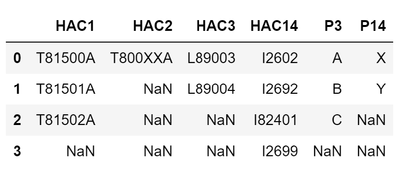df2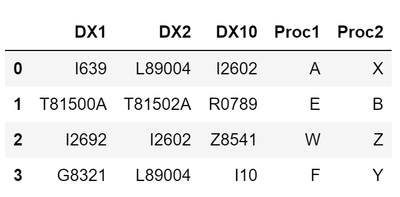I have multiple conditions, if any value from HAC3 is present in DX1-DX10 AND any value from P3 is present in Proc1-Proc2, only then it will be considered, else take it as 0. Similarly, if any value from HAC14 is present in DX1-DX10 AND any value from P14 is present in Proc1-Proc2, only then it will be considered, else take it as 0.

So, the expected output will be: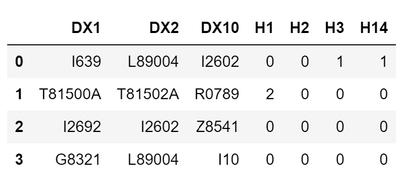The conditions for H1 and H2 remain the same. However, for H3, L89004 from HAC3 is present in first row (DX2) AND A from P3 is present in first row (Proc1), so it is considered as 1. Both the conditions are to be considered simultaneously.

For H14, I2602 from HAC14 is present in first row (DX10) AND X from P14 is present in first row (Proc2), so it is considered as 1.

For the last row, L89004 is present in DX2 but no value from P3 is present in Proc1-Proc2, so it will be 0.

For the last row, Y from P14 is present in Proc2 but no value from HAC14 is present in DX1-DX10, so it will be 0.

Thank you so much for all the help! I really appreciate it!Ksharp
Super User

## Re: Check if the values of multiple columns of one dataset are in multiple columns of another datase

``````/*
You only specify the case of count=1.
What you gonna do if there were two or more values present in DX1-DX10 AND  present in Proc1-Proc2 ?
Or H3 only has value 0 or 1 ?

Check following if it is what you are looking for.
*/
data df1;
input (HAC1 HAC2 HAC3 HAC14 p3 p14) (\$);
cards;
T81500A T800XXA L89003 I2602 A X
T81501A  NaN    L89004 I2692 B Y
T81502A  NaN    NaN    I82401 C NaN
NaN      NaN     NaN   I2699 NaN NaN
;

data df2;
input (DX1 DX2 DX10 proc1 proc2) (\$);
cards;
I639    L89004  I2602 A X
T81500A T81502A R0789 E B
I2692   I2602   Z8541 W Z
G8321   L89004  I10   F Y
;

proc iml;
use df1(keep=HAC:);
read all var _all_ into df1_hac;
close;
use df1(keep=P:);
read all var _all_ into df1_p;
close;
use df2(keep=DX:);
read all var _all_ into df2_dx;
close;
use df2(keep=Proc:);
read all var _all_ into df2_proc;
close;
h1=j(nrow(df2_dx),1,0);
h2=j(nrow(df2_dx),1,0);

h3=j(nrow(df2_dx),1,0);
h14=j(nrow(df2_dx),1,0);

_h3=j(nrow(df2_dx),1,0);
_h14=j(nrow(df2_dx),1,0);

do i=1 to nrow(df2_dx);
h1[i]=sum(element(df2_dx[i,],df1_hac[,1]));
h2[i]=sum(element(df2_dx[i,],df1_hac[,2]));

h3[i] =min( sum(element(df2_dx[i,],df1_hac[,3])) , sum(element(df2_proc[i,],df1_p[,1])) );
h14[i]=min( sum(element(df2_dx[i,],df1_hac[,4])) , sum(element(df2_proc[i,],df1_p[,2])) );

_h3[i] =sum(  element(df2_dx[i,],df1_hac[,3]) , element(df2_proc[i,],df1_p[,1])  );
_h14[i]=sum(  element(df2_dx[i,],df1_hac[,4]) , element(df2_proc[i,],df1_p[,2])  );
end;
create temp var {h1 h2 h3 h14 _h3 _h14};
append;
close;
quit;
data want;
merge df2 temp;
run;``````
Discussion stats
• 16 replies
• 520 views
• 5 likes
• 5 in conversation